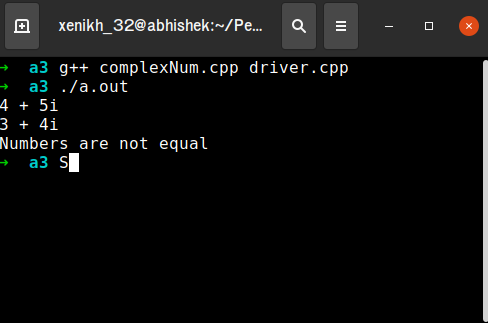# Creating a C++ reusable Header File and its Implementation Files

• Difficulty Level : Medium
• Last Updated : 08 Oct, 2021

Reusability is one of the most important concepts of Software Engineering. Reusability means developing code that can be reused either in the same program or in different programs. C++ allows reusability through inheritance, containership, polymorphism, and genericity. But, there is another way to define independent building blocks. This can be achieved by creating header files and implementation files.

Header files are the files that include the class declaration. The name of the class is generally the same as that of the header file. (For example, a LinkedList class will be stored inside a LinkedList.h header file)

Want to learn from the best curated videos and practice problems, check out the C++ Foundation Course for Basic to Advanced C++ and C++ STL Course for the language and STL. To complete your preparation from learning a language to DS Algo and many more, please refer Complete Interview Preparation Course.

On the other hand, the implementation file consists of the function definition of the class which was defined inside the header file. Generally, the file name is the name of the class, with a .cpp extension. (For example, a LinkedList class’s function definition will be stored inside a LinkedList.cpp header file)

Now, to create an object of the class, defined in the above header file, there must be a main() function. But wait, where to define a main() function, particularly, which file?
The main function is defined inside another file, known as the driver file, or, in some cases, the client file.

Example: Here, complexNum class is implemented. It is split up into two files. The header file has the extension.h and contains the class definitions.

## C++

 `// Header file complexNum.h``#ifndef COMPLEXNUM_H``#define COMPLEXNUM_H` `class` `complexNum {``private``:``    ``int` `real;``    ``int` `imaginary;` `public``:``    ``// With default value,``    ``// default constructor``    ``complexNum(``const` `int` `a = 0,``               ``const` `int` `b = 0);` `    ``// setter function``    ``void` `setNum(``const` `int` `a,``                ``const` `int` `b);` `    ``// Prints the complex number``    ``// in the form real + i(imaginary),``    ``// i->iota``    ``void` `print() ``const``;` `    ``// An overloaded operator to compare``    ``// two complex number objects``    ``bool` `operator==(``const` `complexNum&);``};``#endif`

Implementation File:

## C++

 `// Implementation file``// complexNum.cpp``#include "complexNum.h"``#include ``using` `namespace` `std;` `// A default constructor``complexNum::complexNum(``int` `a,``                       ``int` `b)``{``    ``real = a;``    ``imaginary = b;``}` `// A function to set values``void` `complexNum::setNum(``const` `int` `a,``                        ``const` `int` `b)``{``    ``real = a;``    ``imaginary = b;``}` `// A function to print the complex``// number in the form real +``// (imaginary)i, i->iota``void` `complexNum::print() ``const``{``    ``cout << real << ``" + "` `<< imaginary << ``"i"` `<< endl;``}` `// An overloaded operator to``// compare two complex Number``// objects``bool` `complexNum::operator==(``const` `complexNum& obj)``{``    ``if` `(``this``->real == obj.real && ``this``->imaginary == obj.imaginary) {``        ``return` `true``;``    ``}``    ``return` `false``;``}`

Now to check for correctness and to implement the above complexNum class, there is a need for a driver file. Below is the driver file:

## C++

 `// Driver file to illustrate``// the implementation of``// complexNum.cpp file``#include "complexNum.h"``#include ``using` `namespace` `std;` `// Driver code``int` `main()``{``    ``// Defines a complex number``    ``// object (obj1 = 4 + 5i)``    ``complexNum obj1(4, 5);``    ``complexNum obj2;` `    ``// Defines a complex number``    ``// object (obj2 = 3 + 4i)``    ``obj2.setNum(3, 4);` `    ``// Prints the complex number``    ``obj1.print();``    ``obj2.print();` `    ``// Checks, if two complex``    ``// number objects are equal or``    ``// not``    ``if` `(obj1 == obj2) {``        ``cout << ``"Both the numbers are equal"` `<< endl;``    ``}``    ``else` `{``        ``cout << ``"Numbers are not equal"` `<< endl;``    ``}``    ``return` `0;``}`

Output:Note:
The header, implementation as well as driver files should be in the same folder. Otherwise, provide the link of the present working directory in the include statements.

Header files are already used by programmers, which are very useful, and become handy while implementing various data structures and algorithms. For example, dynamic arrays can be implemented using <vector> header file.

Advantages Of Storing Class Definition In Different Files:

1. Inheritance can be used to achieve code reusability, but the drawback of it is that a class has to be inherited from a class inside the same file. One cannot inherit a class from a different file.
2. But, this issue is resolved by using the header and implementation files, hence making the class reusable.
3. If the class implementation doesn’t change, then there is no need to recompile it.

My Personal Notes arrow_drop_up